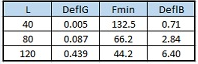# Measurement Error Due to a Bent Ball BarWhere:

L = ball bar length

DeflG = deflection due to gravity

Fmin = minimum force, applied at bar midpoint, to permanently bend the bar

DeflB = deflection at bar midpoint to permanently bend the bar

Assumptions:

tube OD = 0.75 in, tube ID = 0.62 in, steel density = 0.289 lb/in3, steel modulus (E) = 30,000,000 psi, steel yield stress (Sy) = 60,000 psi

Formulas:

DeflG = 5 * density * L ^4 / (24 * E * (OD^2 + ID^2))                         (1)

Fmin = Sy * pi * (OD^4-ID^4)/(8 * OD * L)                                         (2)

DeflB = Sy * L^2 / (6 * OD * E)                                                          (3)

Max measurement error due to a bent ball bar measured at different rotational (about the balls’ common axis) positions:

dx = 20 * density * L^3 * dy / (9 * E * (OD^2 + ID^2))                           (4)

Where:

dx = Max measurement error due to a bent ball bar at different rotational positions

dy = permanent bow measured at midpoint of ball bar

For an 80” ball bar, with 0.1” of permanent bow, and the assumptions stated above:

dx = 20 * 0.289  * 80^3 * 0.1 / (9 * 30000000 * (0.75^2 + 0.62^2))

dx = 0.001158 inches